# 对几种常用贷款进行数据分析

“量化”从字面上可以理解为数量化，如果应用在投资领域上的话，简单的说，就是将每一种投资产品，通过数据分析的方式，将其特点（风险、潜力等等）用详细的数据反映出来，更好地指导投资者获取稳定收益。

1.每月偿还固定金额的贷款（简称等额本息贷款）；

2.先还一笔固定点数（百分比）的贷款，然后每月再以较低的利率还款（简称固定点数贷款）；

3.先以较低的利率还款，满x个月后再以较高的利率还款（简称双利率贷款）。

1.等额本息贷款

月数 | 每月剩余欠款

0 | loan

1 | loan*(1 + r) - X

2 | [loan*(1 + r) - X] * (1 + r) - X => loan*(1+r)^2 - X*(1+r) -X

3 | [loan*(1+r)^2 - X*(1+r) -X]*(1+r) - X => loan*(1+r)^3 - X*(1+r)^2 -X*(1+r) -X

... ...

n | loan*(1+r)^n - X*(1+r)^(n-1) -X*(1+r)^(n-2) - ... -X(1+r)^0

loan*(1+r)^m - X*(1+r)^(m-1) - X*(1+r)^(m-2) - ... - X(1+r)^0 = 0

loan*(1+r)^n - X[(1+r)^0 + (1+r)^1 + ... + (1+r)^(m-1)] = 0

loan*(1+r)^n -X*(1-(1+r)^m) / (-r) = 0

loan*(1+r)^n = X*[(1+r)^m-1]/r

X = loan*r*(1+r)^n/[(1+r)^m-1]

import pylab

import matplotlib

# 指定默认字体

matplotlib.rcParams['font.sans-serif'] = ['SimHei']

matplotlib.rcParams['font.family'] = 'sans-serif'

# 计算在贷款额loan 月利率r和期限m个月下,每月需返还的固定金额

def findPayment(loan, r, m):

return loan * ((r * (1 + r) ** m) / ((1 + r) ** m - 1))

2.等额本金贷款

3.固定点数贷款

4.双利率贷款

class Mortgage(object):

# 构建贷款类, 定义四种贷款都有的属性

def __init__(self, loan, annRate, months):

self.loan = loan # 贷款总额

self.rate = annRate / 12 # 将年利率转化为月利率

self.outstanding = [loan] # 剩余本金

self.months = months # 还款月数

self.paid = [0.0] # 已支付金额

self.legend = None # 贷款描述

self.payment = findPayment(loan, self.rate, months) # 每月还款金额， 默认按照等额本息的方式

def makePayment(self):

# 还款函数，调用此函数进行还款

self.outstanding.append(self.outstanding[-1] - reduction) # 记录剩余本金

self.paid.append(self.payment)

reduction = self.payment - self.outstanding[-1] * self.rate # 还款金额中的本金

def getTotalPaid(self):

# 返回已支付贷款总额

return self.legend

return sum(self.paid)

def __str__(self):

# 返回贷款描述

class Fixed(Mortgage):

# 等额本息子类

# 只需继承Mortgage()，重写描述属性self.legend即可

def __init__(self, loan, r, months):

self.legend = "等额本息, " + str(round(r * 100, 2)) + '%'

Mortgage.__init__(self, loan, r, months)

# 等额本金类贷款每个月要还的本金不变，而利息是随着还款月数的增加而减少的，所以定义一个函数，直接返回一个包含每月还款金额的列表即可

def totalCapitalPayment(loan, months, r):

total = []

for m in range(months):

class FixedCapital(Mortgage):

total.append(loan / months + (loan - m * (loan / months)) * r)

# 等额本金子类

def __init__(self, loan, r, months):

self.total = totalCapitalPayment(loan, months, self.rate)

Mortgage.__init__(self, loan, r, months)

# self.payment =capitalPayment(loan, r, months)

self.capital = loan / months

# 本期剩余本金 = 上期剩余本金 - 每月固定本金

self.legend = "等额本金, " + str(round(r * 100, 2)) + '%'

def makePayment(self, m):

self.paid.append(self.total[m])

# 由于等额本金的每月还款额与当前还款月数相关，所以引入还款月数m

Mortgage.__init__(self, loan, r, months)

self.outstanding.append(self.outstanding[-1] - self.capital)

class FixedWithPts(Mortgage):

# 固定点数子类

self.pts = pts # 固定点数

def __init__(self, loan, r, months, pts):

# 计算第一次按照固定点数还款的金额

def __init__(self, loan, r, months, teaserRate, teaserMonths):

self.paid = [self.loan * (self.pts / 100)]

self.legend = "固定点数, " + str(round(r * 100, 2)) + '%, ' + str(pts) + ' points'

class TwoRate(Mortgage):

# 双利率子类

str(round(r * 100, 2)) + '%'

Mortgage.__init__(self, loan, teaserRate, months)

self.teaserMonths = teaserMonths # 前期低利率月数

self.nextRate = r / 12 # 后期高利率

self.teaserRate = teaserRate # 前期低利率

self.legend = '双利率, ' + str(teaserRate * 100) + '% for ' + str(self.teaserMonths) + ' months, then ' + \

def makePayment(self):

Mortgage.makePayment(self) # 未到达teaserMonths时，每月的还款金额

# 如果到达teaserMonths，则使用self.nextRate高利率，后面每月的付款金额按照剩余本金、利率和月数重新计算

if len(self.paid) - 1 == self.teaserMonths:

self.payment = findPayment(self.outstanding[-1], self.rate, self.months - self.teaserMonths)

self.rate = self.nextRate

def plotPayments(self, style):

# 统计每月还款额

pylab.plot(self.paid[1:], style, label=self.legend)

def plotTotPd(self, style):

# 统计每月还款总额

totPd.append(totPd[-1] + self.paid[i])

totPd = [self.paid]

for i in range(len(self.paid)):

def plotBalance(self, style):

pylab.plot(totPd, style, label=self.legend)

# 统计每月剩余本金

totPd = [self.paid]

pylab.plot(self.outstanding, style, label=self.legend)

def plotNet(self, style):

# 统计每月支付总利息

equityAcquired = pylab.array([self.loan] * len(self.outstanding)) - pylab.array(self.outstanding)

for i in range(1, len(self.paid)):

totPd.append(totPd[-1] + self.paid[i])

# 先通过数组计算出每月偿还的本金（贷款总额 - 每月剩余本金）

pylab.plot(net, style, label=self.legend)

# 再用每月的总还款额 - 每月偿还本金 = 每月偿还利息

net = pylab.array(totPd) - equityAcquired

def plotMortgages(morts, amt):

def labelPlot(figure, title, xLabel, yLabel):

pylab.figure(figure) # 指定当前图，即绘制前要先指定图的figure值

pylab.title(title) # 设置标题

pylab.ylabel(yLabel) # 设置y轴标签

pylab.xlabel(xLabel) # 设置x轴标签

styles = ['k-', 'k-.', 'k:', 'b-'] # 设置各类贷款对应的曲线样式

pylab.legend(loc='best') # 将描述信息放在不与曲线冲突的最合适区域

for i in range(len(morts)):

payments, cost, balance, netCost = 0, 1, 2, 3 # 设置图的figure，对各类曲线按照指标分类

morts[i].plotTotPd(styles[i]) # 总还款额

pylab.figure(payments) # 根据figure值，将各类曲线绘制到对应的图中

morts[i].plotPayments(styles[i]) # 月还款额

morts[i].plotNet(styles[i]) # 月支付利息

pylab.figure(cost)

pylab.figure(balance)

morts[i].plotBalance(styles[i]) # 剩余本金

pylab.figure(netCost)

labelPlot(balance, '贷款' + str(amt) + '元的每月本金剩余情况', '月数', '剩余未还本金')

labelPlot(payments, '贷款' + str(amt) + '元的每月还款情况', '月数', '月还款金额')

labelPlot(cost, '贷款' + str(amt) + '元的还款总额', '月数', '已支付金额')

labelPlot(netCost, '贷款' + str(amt) + '元的累计利息支付情况', '月数', '支付的累计利息')

def compareMortgages(amt, years, fixedRate, pts, ptsRate, lowRate, highRate, lowMonths):

# 比较各类贷款的总还款额

totMonths = years * 12

fixed1 = Fixed(amt, fixedRate, totMonths)

fixed3 = FixedWithPts(amt, ptsRate, totMonths, pts)

fixed2 = FixedCapital(amt, fixedRate, totMonths)

twoRate = TwoRate(amt, highRate, totMonths, lowRate, lowMonths)

fixed2.makePayment(m) # 单独调用包含参数m的等额本金还款参数

morts = [fixed1, fixed3, twoRate] # 先对除等额本金外的其他三类贷款进行还款

for m in range(totMonths):

for mort in morts:
morts.insert(1, fixed2) # 还款完毕后再加入贷款列表

mort.makePayment()

pylab.show()

# 展示四种贷款方式各自的还款总额

# for m in morts:

# print(" Total payments = $" + str(int(m.getTotalPaid()))) # print(m) plotMortgages(morts, amt) # 带入实际的值进行比较： compareMortgages(200000, 30, 0.07, 3.25, 0.05, 0.045, 0.095, 48) # 比较结果 Fixed, 7.0% Total payments =$479017

Fixed Capital, 7.0%

Total payments = $393011 Total payments =$410583

Fixed, 5.0%, 3.25 points

4.5% for 48 months, then 9.5%

Total payments = \$551444

1.每月还款额的变化：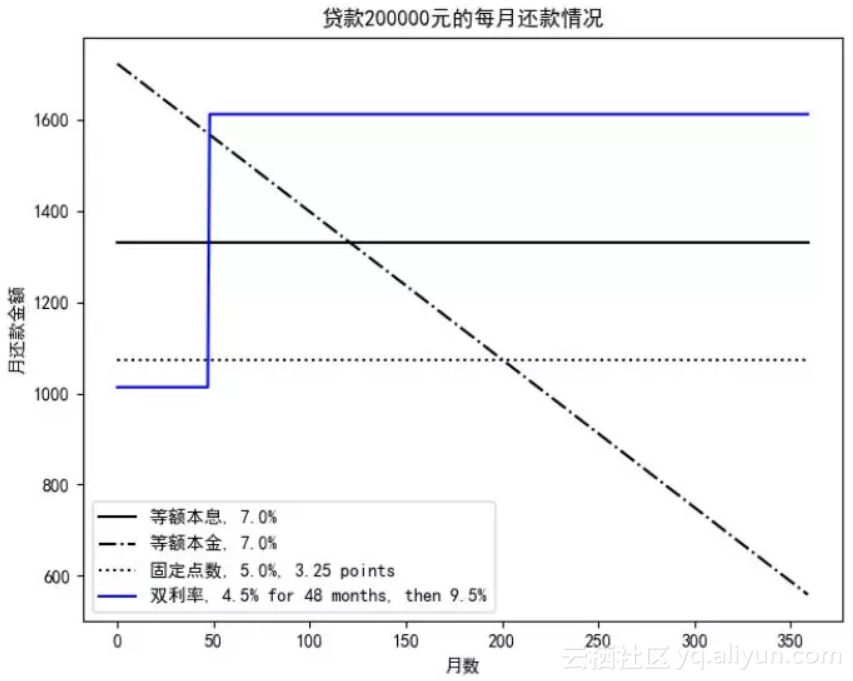2.整个贷款周期的本金+利息总和：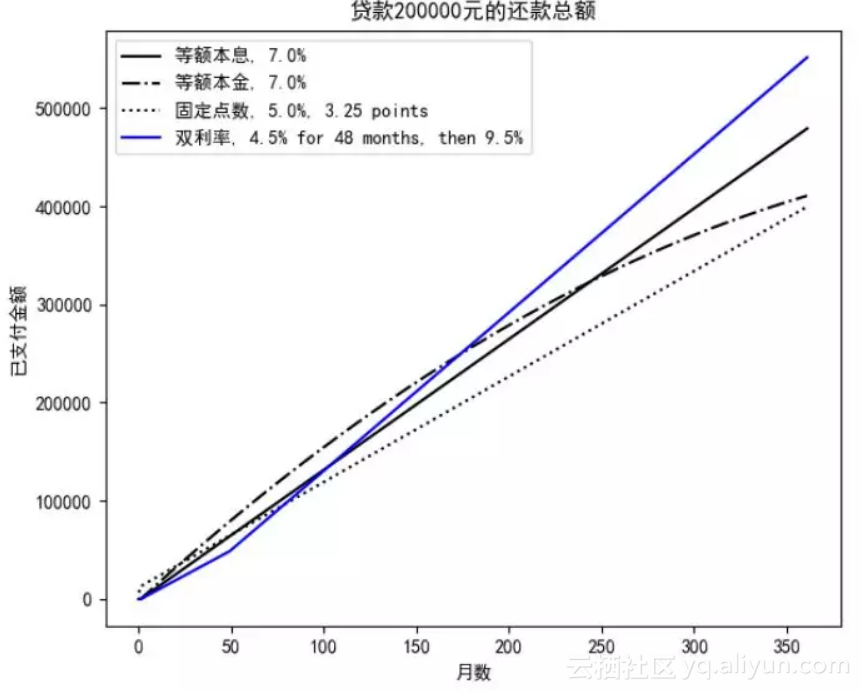3.剩余未还本金的情况：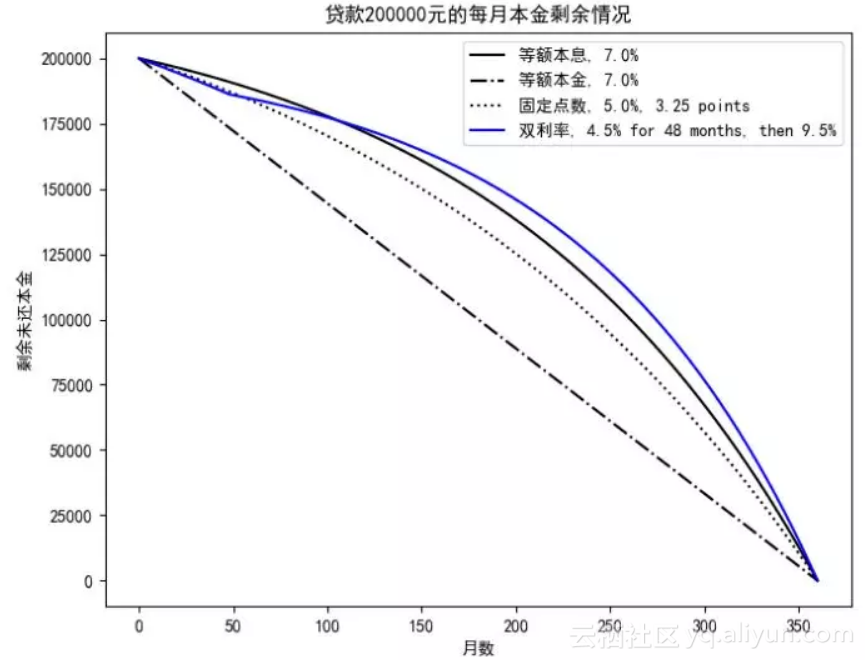4.每月累计支付的利息情况：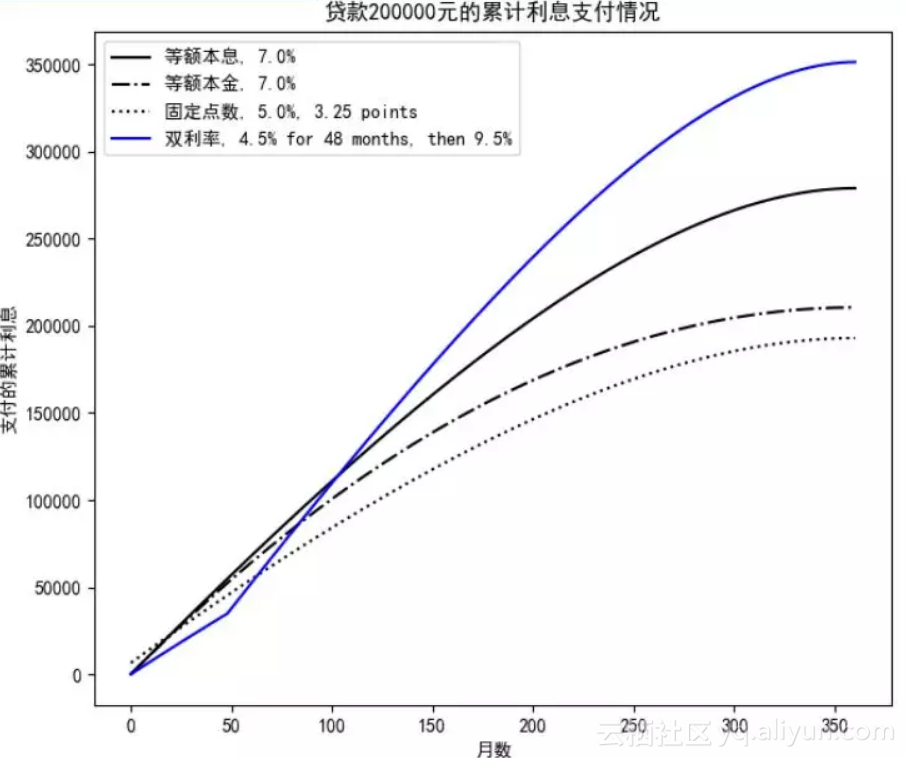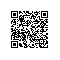Python爱好者使用钉钉扫一扫加入圈子
+ 订阅